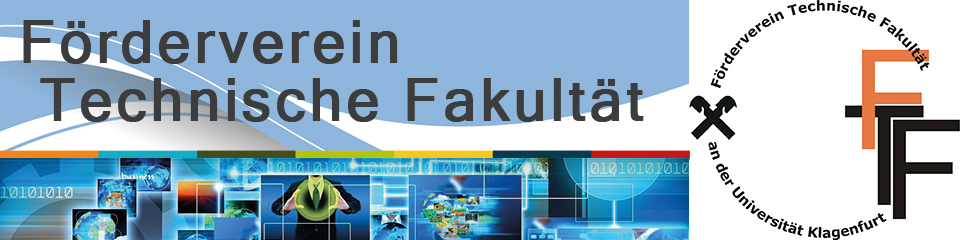## MULTIHARMONIC FINITE ELEMENT SOLVERS

The talk is devoted to fast iterative solvers for frequency-domain finite element equations approximating linear and nonlinear parabolic initial boundary value problems as well as eddy current problems arising in electromagnetics with time-harmonic excitations. Switching from the time domain to the frequency domain allows us to replace the expensive time integration procedure by the solution of a simple

linear elliptic system for the amplitudes belonging to the sine- and to the cosine-excitation or a large nonlinear elliptic system for the Fourier coefficients in the linear and nonlinear case, respectively. The fast solution of the corresponding linear and nonlinear system of finite element equations is crucial for the competitiveness of this method in comparison with standard time integration methods.

zp8497586rq